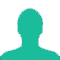Narayan spends 30% of his income on education and 50% of the remaining on food. He gives Rs. 1000 as monthly rent and now has Rs. 1800 left with him. What is his monthly income?

A. Rs. 8000

B. Rs. 7000

C. Rs. 9000

D. Rs. 6000

Solution(By Examveda Team)

Narayan's saving and rent = 1000 + 1800 = Rs. 2800
Let his monthly income be Rs. 100
30% of his income he spent on education i.e. Rs. 30
Remaining = 100 - 30 = 70
50% of remaining on food = $$\frac{{70 \times 50}}{{100}} = {\text{Rs}}{\text{. 35}}$$
Now, that 35 must be equal to his saving and rent i.e.
35 = 2800 then,
1 = $$\frac{{2800}}{{35}}$$
100 = $$\frac{{2800 \times 100}}{{35}} = {\text{Rs}}{\text{. 8000}}$$
So, his income = Rs. 8000

1.solution:
assume total income=X
X - (30% of X) - (50 % of (X- 30% of X)) - 1000=2800
by solving this we get ,
X=8000

2.solution:
assume total income=X
X- 30% of X- 50% of X-1000=1800
by solving this we get
X=13000.

((13000-30% of 13000- 50% of 13000-1000=1800))

3.check option method
a} 30% of 8000=2400
8000-2400=5600
50% of 5600= 2800
8000-(2400+2800+1000)=1800
this condition is true then 8000 is right ans

4.i can't understand

Related Questions on Percentage